# 1. When mixed, solutions of silver nitrate, AgNO, and sodium phosphate, Na3PO4, will form a precipitate...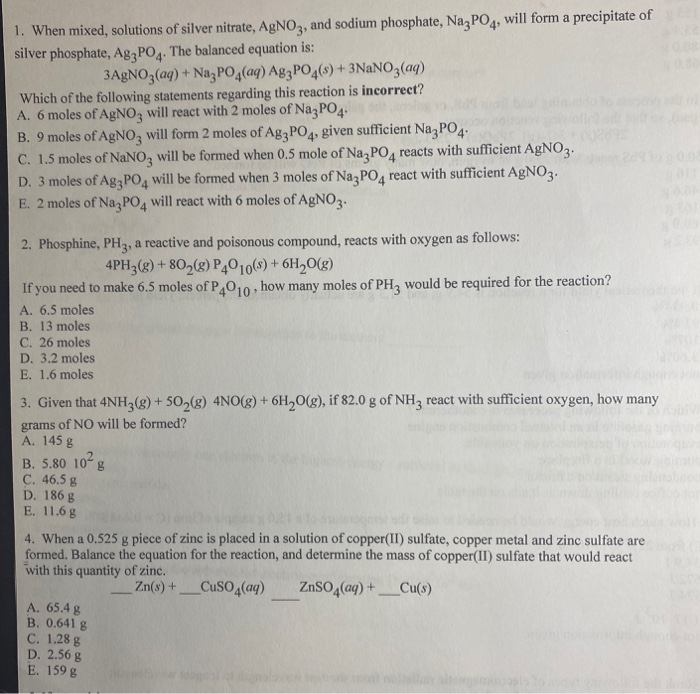1. When mixed, solutions of silver nitrate, AgNO, and sodium phosphate, Na3PO4, will form a precipitate of silver phosphate, Ag2PO4. The balanced equation is: 3AgNO3(aq) + Na3PO4(aq) Ag3PO4(s) + 3NaNO3(aq) Which of the following statements regarding this reaction is incorrect? A. 6 moles of AgNO, will react with 2 moles of Na2PO4 B. 9 moles of AgNO3 will form 2 moles of Ag3PO4, given sufficient Na3PO4 C. 1.5 moles of NaNO, will be formed when 0.5 mole of Na PO, reacts with sufficient AgNO. D. 3 moles of Ag3PO4 will be formed when 3 moles of Na PO, react with sufficient AgNO3. E. 2 moles of Na PO, will react with 6 moles of AgNO. 2. Phosphine, PH2, a reactive and poisonous compound, reacts with oxygen as follows: 4PH3(g) +802(8) P4010(s) + 6H20(g) If you need to make 6.5 moles of P,010, how many moles of PH, would be required for the reaction? A. 6.5 moles B. 13 moles C. 26 moles D. 3.2 moles E. 1.6 moles 3. Given that 4NH3(g) + 50 (g) 4NO(g) + 6H2O(g), if 82.0 g of NH3 react with sufficient oxygen, how many grams of NO will be formed? A. 145 g B. 5.80 1028 C. 46.5 g D. 1868 E. 11.68 4. When a 0.525 g piece of zinc is placed in a solution of copper(II) sulfate, copper metal and zinc sulfate are formed. Balance the equation for the reaction, and determine the mass of copper(II) sulfate that would react with this quantity of zinc. - Zn(s) + __CuSO4(aq) ZnSO4(aq) + _Cu(s) A. 65.4 g B. 0.641 g C. 1.28 g D. 2.56 g E. 159 g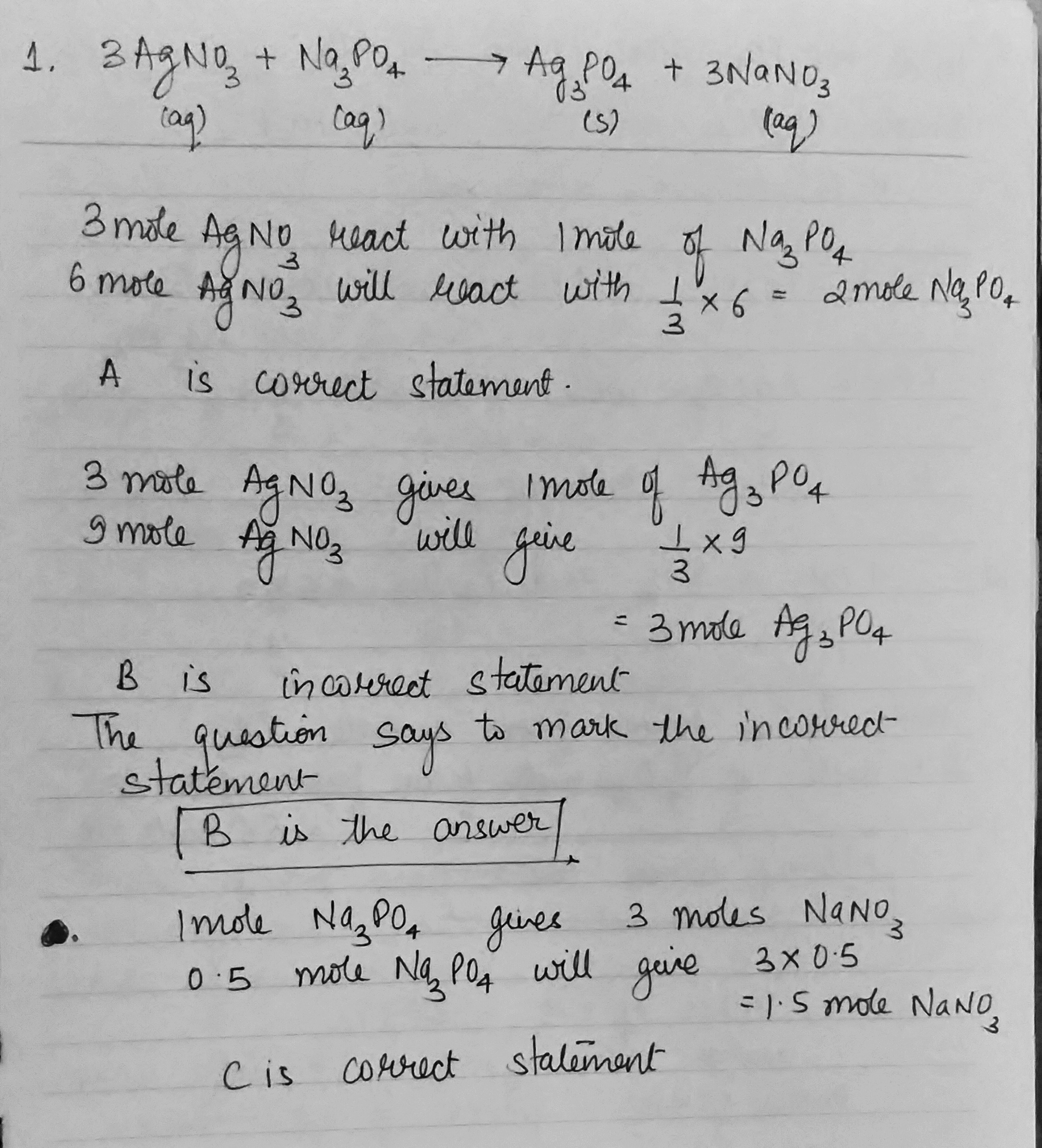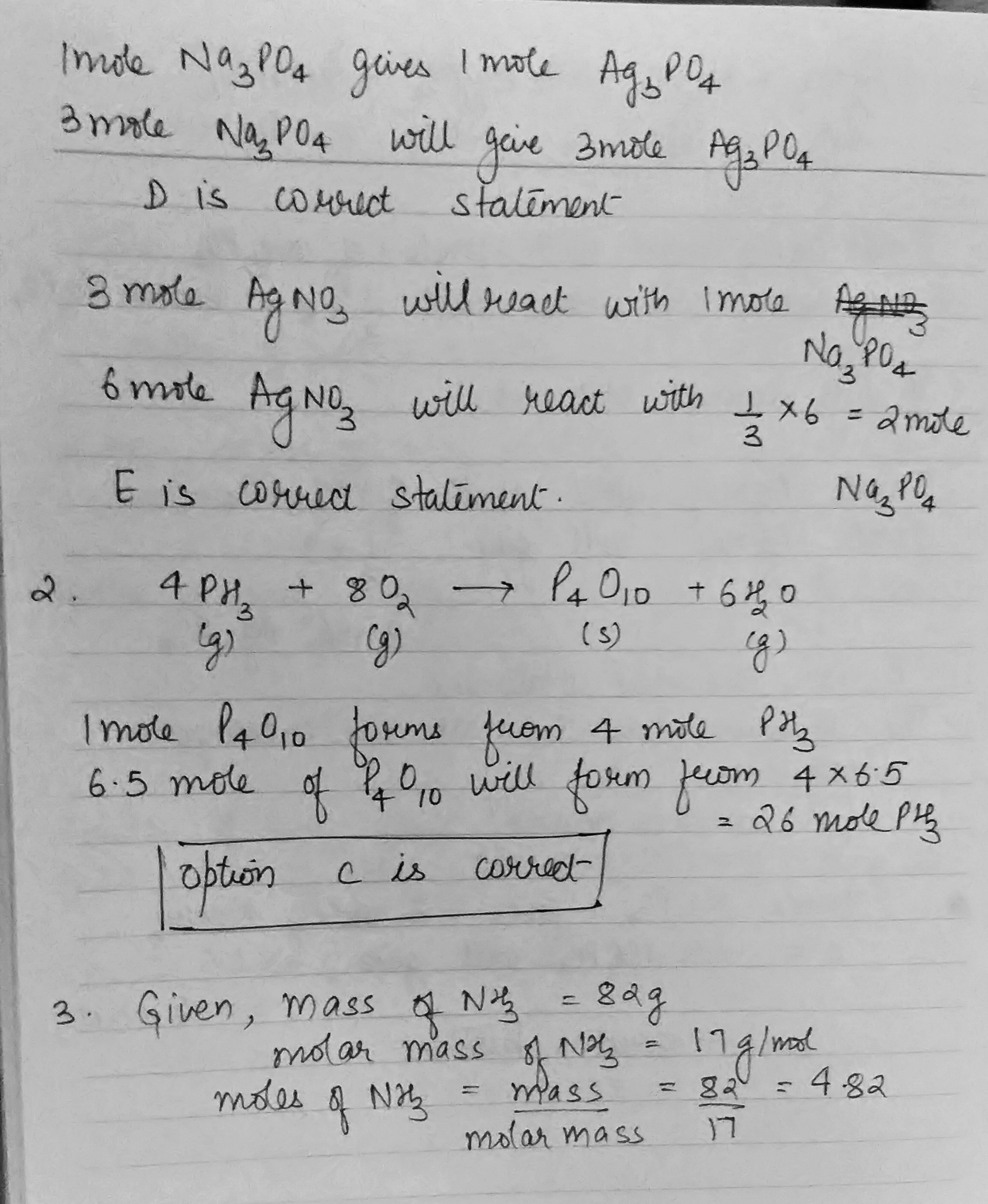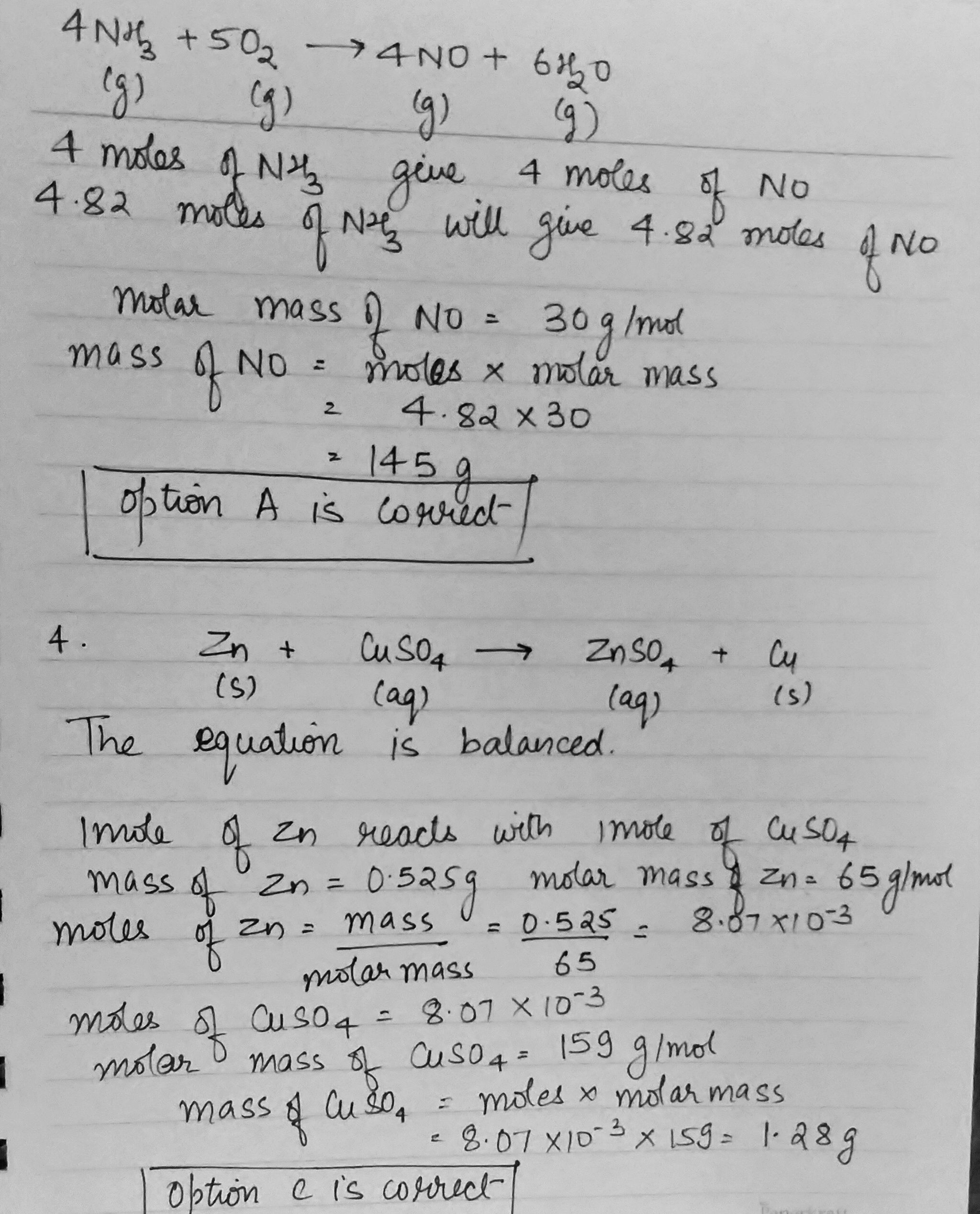#### Earn Coin

Coins can be redeemed for fabulous gifts.

Similar Homework Help Questions
• ### Double Replacement potassium carbonate (aq) + silver nitrate (aq) → potassium nitrate (aq) + silver carbonate...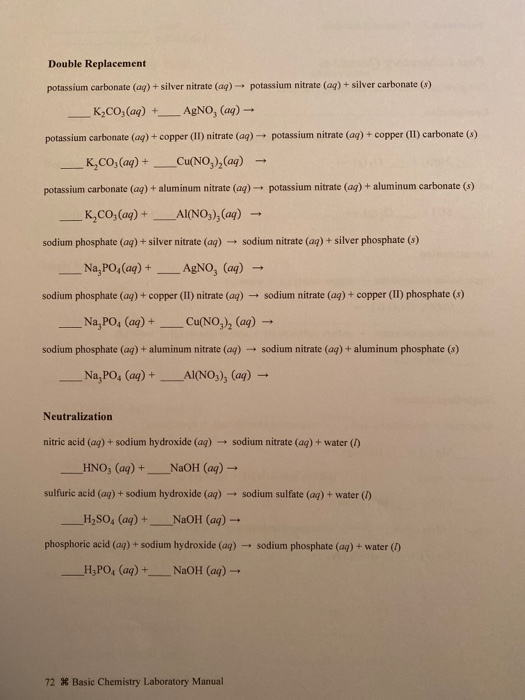Double Replacement potassium carbonate (aq) + silver nitrate (aq) → potassium nitrate (aq) + silver carbonate (s) K2CO3(aq) + AgNO, (ag) - potassium carbonate (aq) + copper (II) nitrate (aq) potassium nitrate (aq) + copper (II) carbonate (8) K,CO, (aq) + _Cu(NO, ),(aq) potassium carbonate (aq) + aluminum nitrate (aq) + potassium nitrate (aq) + aluminum carbonate (3) K.CO; (aq) + _Al(NO3) (aq) - sodium phosphate (aq) + silver nitrate (aq) sodium nitrate (aq) + silver phosphate (8) Na, PO...

• ### 6. (16 points) Magnesium bromide reacts with silver nitrate to form silver bromide (solid) and magnesium...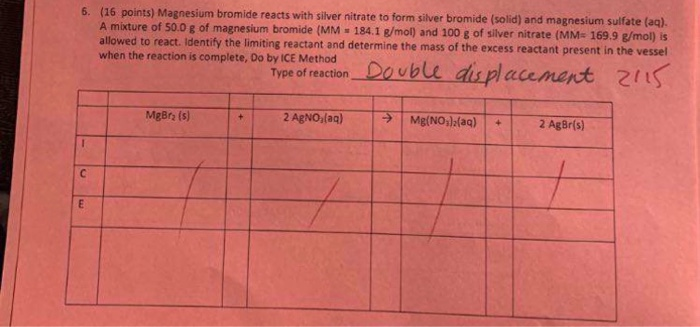6. (16 points) Magnesium bromide reacts with silver nitrate to form silver bromide (solid) and magnesium sulfate (aq). A mixture of 50.0 8 of magnesium bromide (MM 184.1 g/mol) and 100 g of silver nitrate (MM- 169.9 g/mol) is allowed to react. Identify the limiting reactant and determine the mass of the excess reactant present in the vessel when the reaction is complete, Do by ICE Method Type of reaction Double displacement 2015 MgBra (5) + 2 AgNO,(aq) > Mg(NO)2(aq)...

• ### 6. (16 points) Magnesium bromide reacts with silver nitrate to form silver bromide (solid) and magnesium sulfate (a...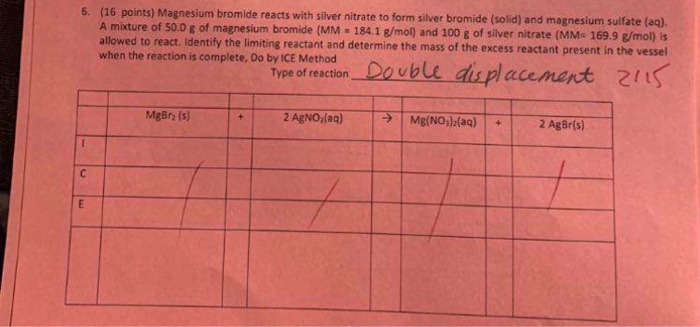6. (16 points) Magnesium bromide reacts with silver nitrate to form silver bromide (solid) and magnesium sulfate (aq). A mixture of 50.0 g of magnesium bromide (MM - 184.1 g/mol) and 100 g of silver nitrate (MM- 169.9 g/mol) is allowed to react. Identify the limiting reactant and determine the mass of the excess reactant present in the vessel when the reaction is complete, Do by ICE Method Type of reactionDouble displacement 2115 MgBra (s) 2 AgNO (aq) > Mg(NO3)2(aq)...

• ### all questions please. thank you. 1. Aqueous solutions of silver nitrate and sodium sulfate are mixed....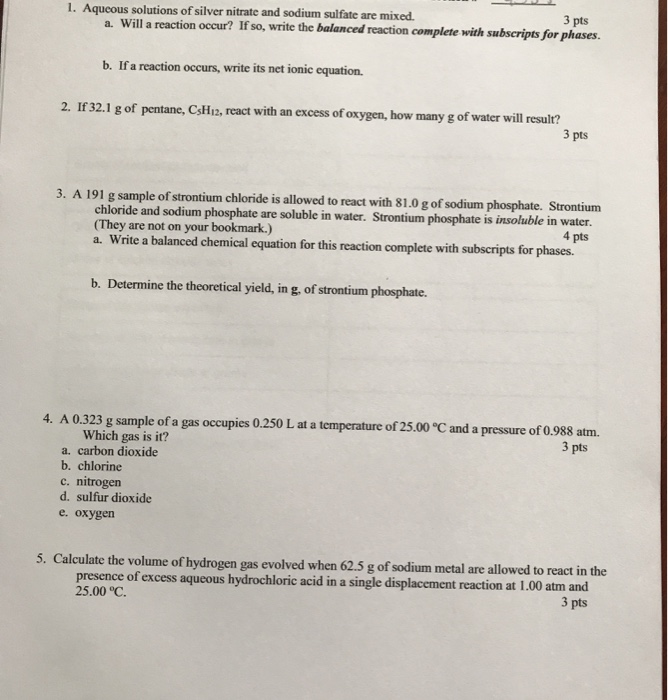all questions please. thank you. 1. Aqueous solutions of silver nitrate and sodium sulfate are mixed. 3 pts a. Will a reaction occur? If so, write the balanced reaction complete with subscripts for phases. b. If a reaction occurs, write its net ionic equation. 2. If 32.1 g of pentane, CsH12, react with an excess of oxygen, how many g of water will result? 3 pts 3. A 191 g sample of strontium chloride is allowed to react with 81.0...

• ### Use the References to access impertant valers if needed fer this question. Visited When silver nitrate...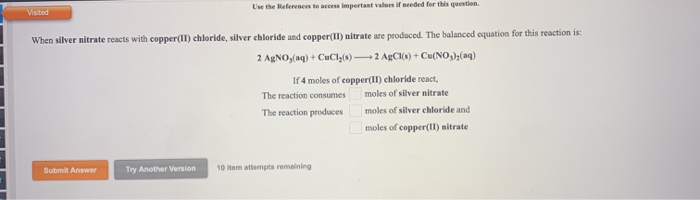Use the References to access impertant valers if needed fer this question. Visited When silver nitrate reacts with copper(II) chloride, silver chloride and copper(II) nitrate are produced. The balanced equation for this reaction is: 2 AgNOy(aq)+ CuCly(s)2 AgCIs) + Cu(NO)(aq) If 4 moles of copper(II) chloride react, moles of silver nitrate The reaction consumes moles of silver chloride and The reaction produces moles of copper(1) nitrate 10 item attempts remaining Try Another Version Submit Answer Chapter 10: EOC HW Use...

• ### Experiment 8 Double Replacement Reactions Background: Some reactions have the net effect of causing the cation...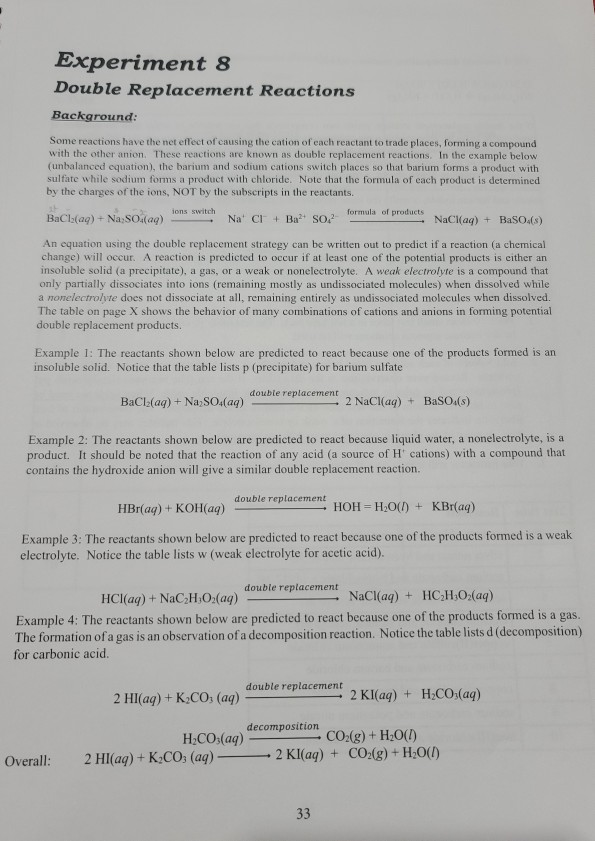Experiment 8 Double Replacement Reactions Background: Some reactions have the net effect of causing the cation of each reactant to trade places, forming a compound with the other anion. These reactions are known as double replacement reactions. In the example below (unbalanced equation), the barium and sodium cations switch places so that barium forms a product with sulfate while sodium forms a product with chloride. Note that the formula of each product is determined by the charges of the ions,...

• ### When you write the answers, please write the page number for which the question is located....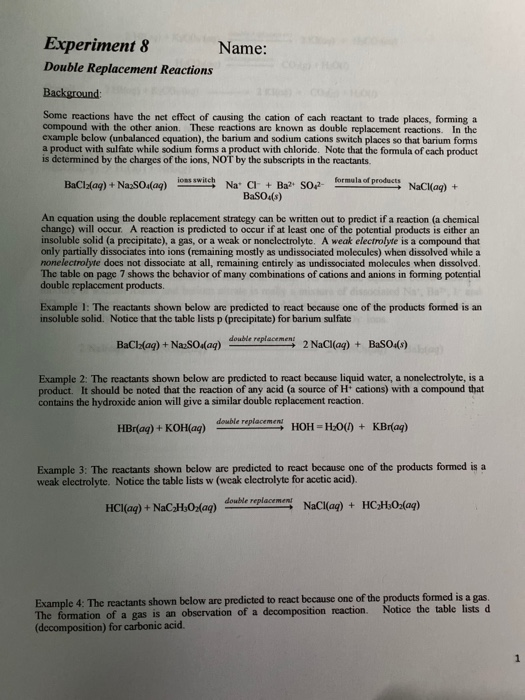When you write the answers, please write the page number for which the question is located. Thank you! Experiment 8 Name: Double Replacement Reactions Background: Some reactions have the net effect of causing the cation of cach reactant to trade places, forming a compound with the other anion. These reactions are known as double replacement reactions. In the example below (unbalanced equation), the barium and sodium cations switch places so that barium forms a product with sulfate while sodium forms...

• ### Sample Chp. 3 & 4 Problems Name 1. Aqueous solutions of sodium hypochlorite (NaOCI), best known...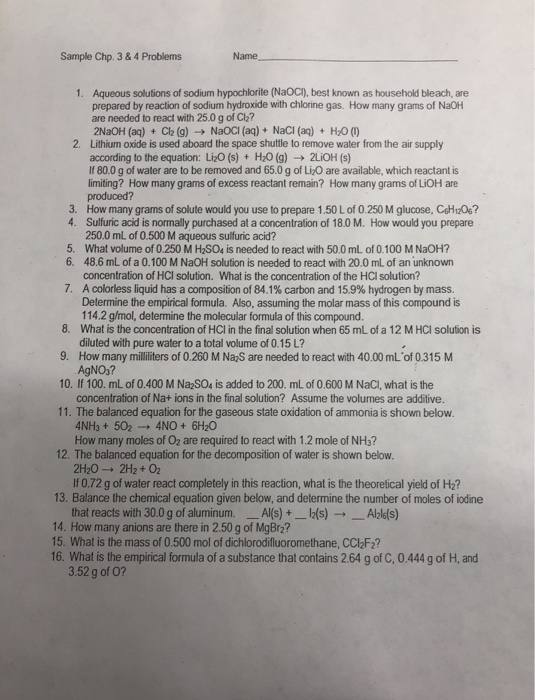Sample Chp. 3 & 4 Problems Name 1. Aqueous solutions of sodium hypochlorite (NaOCI), best known as household bleach, are prepared by reaction of sodium hydroxide with chlorine gas. How many grams of NaOH are needed to react with 25.0 g of Cl? 2NaOH(aq) + Cl2 (9) NaOCI (aq) + NaCl (aq) + H2O (U) 2. Lithium oxide is used aboard the space shuttle to remove water from the air supply according to the equation: Li20 (s) + H20 (9)...

• ### 1.   In both the sodium iodide test and the silver nitrate test, why does 2-bromobutane react faster...1.   In both the sodium iodide test and the silver nitrate test, why does 2-bromobutane react faster than 2-chlorobutane? Bromine is a better leaving group since it is a weaker base than chlorine is. 2.   a. Why does benzyl chloride react under both SN1 and SN2 conditions?             Benzyl chloride is a primary alkyl halide, hence reactive under SN2 conditions.             The primary carbocation formed due to the departure of Cl- is stabilized by the pi electrons in the benzene ring.         b. Why is...

• ### QUESTION 1 Consider the reaction 2Fe(s) + 30,(€) ► Fe2O3(s) If 13.6 g of iron(III) oxide...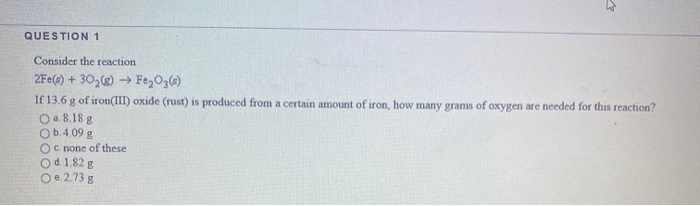QUESTION 1 Consider the reaction 2Fe(s) + 30,(€) ► Fe2O3(s) If 13.6 g of iron(III) oxide (rust) is produced from a certain amount of iron, how many grams of oxygen are needed for this reaction? O a. 8.18 g b.4.09 g O c. none of these O d. 1.82 g O e 2.73 g QUESTION 2 The balanced equation 2Cu(s) + O2(g) → 2CuO (8) tells us that 8.0 mol of Cu O a must react with 256 g of...